## Tuesday, June 28, 2016

### Fantasy football

From this post:

I have been working on a hobby project for a while that involves finding all possible team rosters given the constraints of a salary cap and player positions while trying to N teams with the maximum projection. This is specifically for fantasy football, but the problem can be more generalized to a maximization problem. I can't find a more efficient solution than using nested for loops. I am using python dictionaries to track specific player data by position where qbs[playerName] = {Salary: X, Projection: Y} and rbs[playerName] = {Salary: X, Projection: Y}, etc.

The constraints are as follows:

• 1 quarterback (qb)
• 2 running backs (rb1, rb2)
• 3 wide receivers (wr1, wr2, wr3)
• 1 tight end (te)
• 1 defense (dst)
• 1 flex player (can be a running back, a tight end, or a wide receiver)
• total salary has to be <= N

I assume the two N’s in this description are different: one is the salary cap, the other is the number of solutions to be found. Let’s try to generate some random data: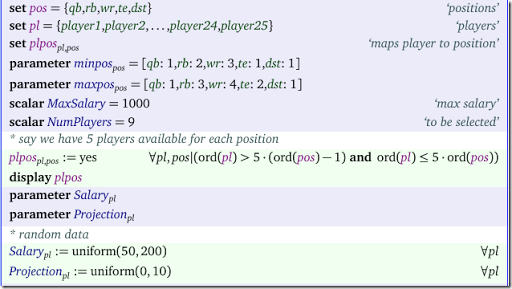I just generate 25 candidate players: 5 candidate players for each 5 positions. This is stored in the set $$plpos_{pl,pos}$$.

The basic model for one best solution is as follows: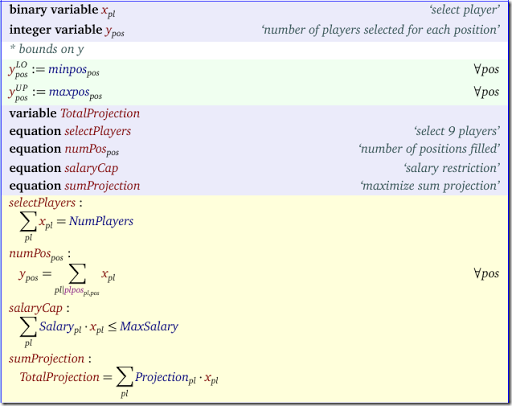Note that we don’t strictly need the $$y$$ variables. We can substitute them out (or relax them to continuous variables).

I assume we want to generate 10 solutions (see set $$sols$$ below). The idea to find the 10 best solutions is to implement the following algorithm:

1. Solve MIP
2. If enough solutions: STOP
3. Add a constraint (cut) to the model that forbids the current solution
4. Go to step 1.

In the code below the cuts are expressed in equation $$cut_{sol}$$, where $$sol$$ is a dynamic set that grows each iteration. We start with the empty set: $$sol:=\emptyset$$.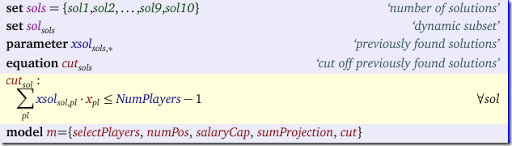Usually the cut to prevent an earlier found binary solution vector $$x^*_i$$ is as follows:

$\sum_{i|x^*_i=1} x_i -\sum_{i|x^*_i=0} x_i \le n-1$

where $$n$$ is the number of $$x^*_i=1$$. For a derivation see here. In our case we know $$\sum x_i=n$$ so we can use a simpler cut:

$\sum_{i|x^*_i=1} x_i \le n-1$

The algorithm looks like: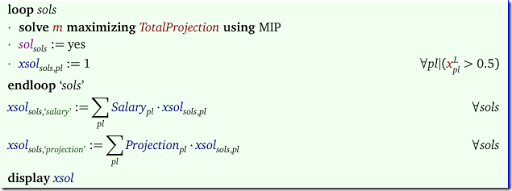Because in integer programming we can see solution values like 0.99999, we just consider all values $$>0.5$$ as one. When we run this code we see: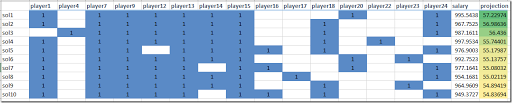All 10 solutions obey the salary cap. The total projection (the objective) is slowly getting worse when we search for new solutions.

It is noted that some solvers provide tools to generate “next best” solutions more efficiently (e.g. Cplex’s solution pool).

I was pointed towards a related approach documented in this paper (1). I did not read it: the publisher charges $50 to download the pdf file (for 13 pages that is$3.85 per page, beating this paper).

#### References

(1) Andrew C. Trapp and Renata A. Konrad, Finding diverse optima and near-optima to binary integer programs, IIE Transactions, 47 (11), 2015, pp.1300-1312.